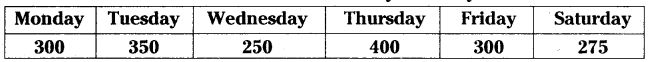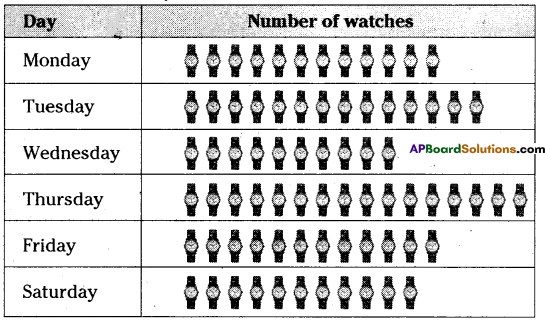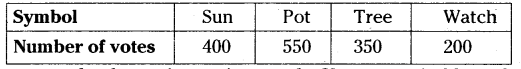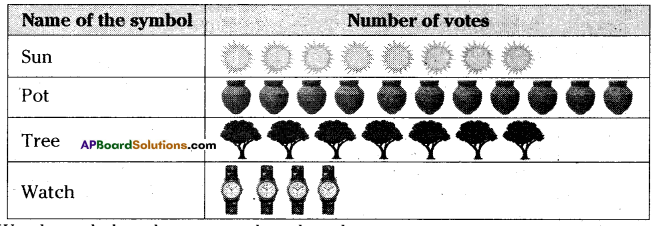AP State Syllabus AP Board 6th Class Maths Solutions Chapter 12 Data Handling Ex 12.2 Textbook Questions and Answers.

## AP State Syllabus 6th Class Maths Solutions 12th Lesson Data Handling Ex 12.2Question 1.
The number of wrist watches as manufactured by a factory in a week are as fallows.Represent the data using a pictograph. Choose a suitable scale.
Solution:
GCD of the given numbers is 25.
So, Scale : 1 watch symbol = 25 watches.Note:
To represent 300 watches. $$\frac{300}{25}$$ = 12 symbols.
To represent 350 watches, $$\frac{350}{25}$$ = 14 symbols.
To represent 250 watches, $$\frac{250}{25}$$ = 10 symbols.
To represent 400 watches, $$\frac{400}{25}$$ = 16 symbols.
To represent 400 watches, $$\frac{300}{25}$$ = 12 symbols.
To represent 400 watches, $$\frac{275}{25}$$ = 11 symbols.Question 2.
Votes polled for various candidates in a Sarpanch election are shown below, against their symbols in the following table.Represent the data using a pictograph. Choose a suitable scale. Answer the following questions:
(i) Which symbol got least votes?
(ii) Which symbol candidate won in the election?
Solution:
GCD or HCF of the given numbers is 50.
So, scale : 1 symbol : 50 votes
To represent 400 votes, $$\frac{400}{50}$$ = 8 symbols of Sun.
To represent 550 votes, $$\frac{550}{50}$$= 11 symbols of Pot.
To represent 350 votes, $$\frac{350}{50}$$= 7 symbols of Tree.
To represent 200 votes, $$\frac{200}{50}$$ = 4 symbols of Watchi) Watch symbol got least votes than the other.
ii) Among all symbols pot symbol got more votes that is 550. So, Pot symbol candidate won in the election.